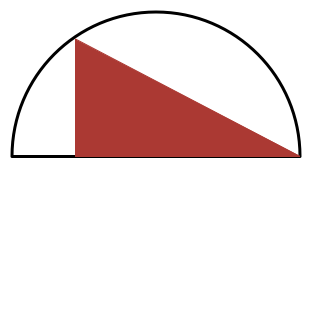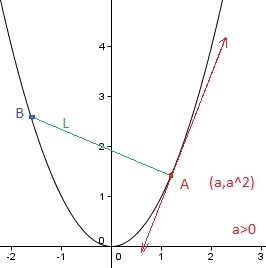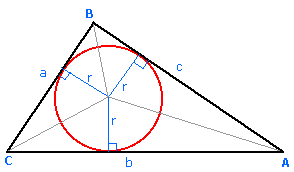Calculus

# Extrema: Level 3 ChallengesA circle rests in the interior of the parabola with equation $$y=x^2$$ so that it is tangent to the parabola at two points. How much higher is the center of the circle than the points of tangency?

Source: Mandelbrot #2Let $$S$$ be the maximum possible area of a right triangle that can be drawn in a semi-circle of radius $$1$$, where one of the legs (and not the hypotenuse) of the triangle must lie on the diameter of the semicircle.

If $$S = \dfrac{a\sqrt{b}}{c},$$ where $$a,c$$ are positive coprime integers and $$b$$ is a positive square-free integer, find $$a + b + c.$$

How many real values of $$x$$ satisfy the equation

$\large {x}^{2}-{2}^{x}=0?$Suppose that two people, A and B, walk along the parabola $$y=x^2$$ in such a way that the line segment $$L$$ between them is always perpendicular to the line tangent to the parabola at A's position $$(a,a^2)$$ with $$a > 0$$. If B's position is $$(b,b^2)$$, what value of $$b$$ minimizes $$L$$?What is the $$least$$ $$perimeter$$ of an isosceles triangle in which a circle of radius $$\sqrt{3}$$ can be inscribed ?

Note : The picture shown is a rough one.

×

Problem Loading...

Note Loading...

Set Loading...